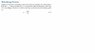# Work Energy Theorem: Explaining Constant Force and Acceleration

• Miike012

#### Miike012

I posted a picture of the paragraph that I am confused about...

The following paragraph says the body accelerates even though the forces are constant...

can some one explain why the acceleration is not zero?

#### Attachments

•Acceleration.jpg
7.9 KB · Views: 368
Because the total forces don't equate to zero. Think about a car driving down the road(x), and you have a crosswind pushing you to the left(y). the cross wind doesn't affect the x distance. In this case your F1, F2, and F3 add together to create a constant of sigma F

Remember Newton's laws an object in motion will stay in motion, less something acts upon it to accelerate, or slow down said object. Acceleration can be positive, or negative.

Your force would have to equal out to zero for the acceleration to equal zero. Force is defined as Acceleration * mass

F=m*a getting acceleration by itself would equal F/m,or you could go for finding the mass = F/a